# Class&Instance¶

## Class¶

# 不带继承的类
class ClsName:    # 类名
# 静态字段，通过类访问 print(ClsName.name), 在内存中只保存一份
name = ''
age = 0        # public类变量，任何区域都能访问
_weight = 0    # protected类变量，当前类和子类和同一模块中才能访问
__height = 0   # private类变量，只有当前类才能访问

# 构造方法
def __init__(self,[para1[, para2[, paraN]):
""" 这里是类文档 """
# 普通字段，通过实例来访问 print(clsInstance.para1), 每个实例中都保存
self.para1 = para1
self.para2 = para2

# 类方法
def method(self):
""" 这里是方法注释 """
<statement>

# 类的私有方法
def __private_method(self):
<statement>

# 继承了别的类的类
class ClsName(BaseClsName1[, Base2[, Base3[, BaseN]):    # 括号内是这个类所继承的父类
<statement>


### 类变量 VS 对象变量¶

#### 差别¶

class Person:
# 类变量、静态字段、类属性
eyes = 2
nose = 1

def __init__(self, name, age):
# 对象变量、普通字段、实例属性
self.name = name
self.age = age

![](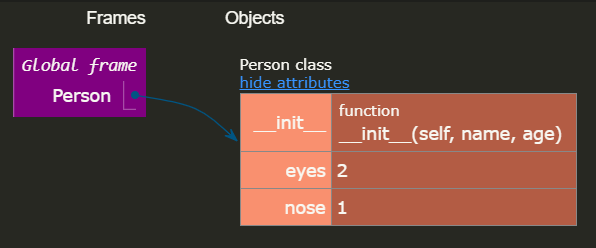# 创建两个对象（实例化）
p1 = Person('Boii', 20)
p2 = Person('Cai', 18)

# 访问静态变量
print(Person.eyes)    # 2 通过类名访问
print(p1.eyes)        # 2 通过对象访问
print(p2.nose)        # 1 通过对象访问

# 访问对象变量
print(p1.name)   # Boii
print(p2.name)   # Cai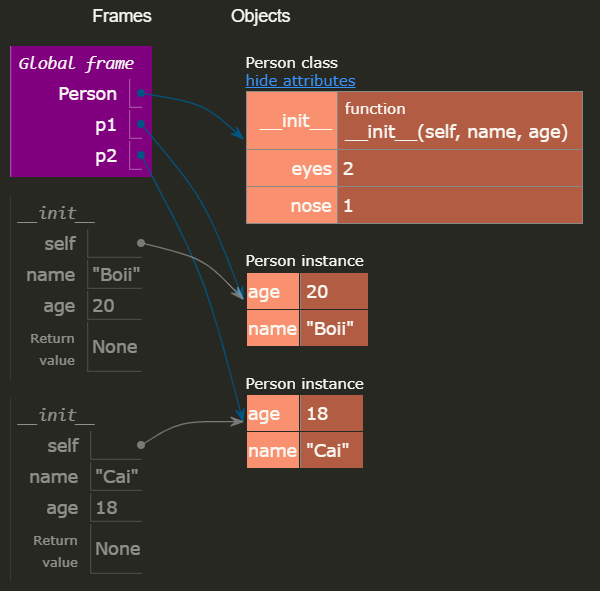# 修改静态变量
Person.eyes = 1

print(p1.eyes)    # 1
print(p2.eyes)    # 1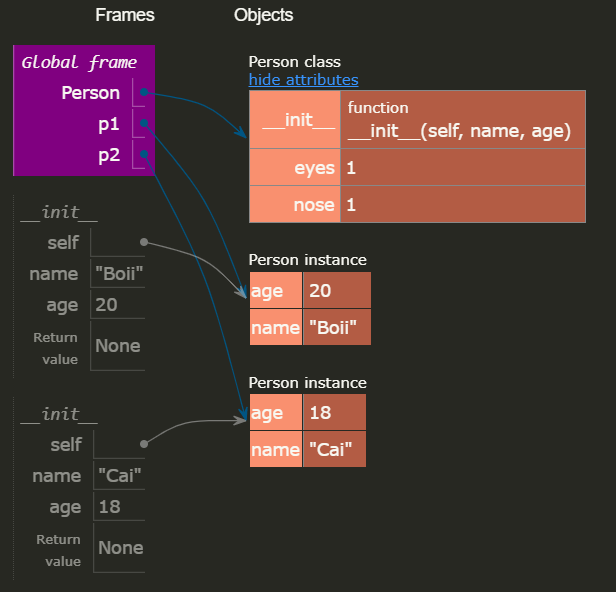# 修改对象变量
p1.age = 50

print(p1.age)    # 50
print(p2.age)    # 18, 改了对象A的，对象B是不受影响的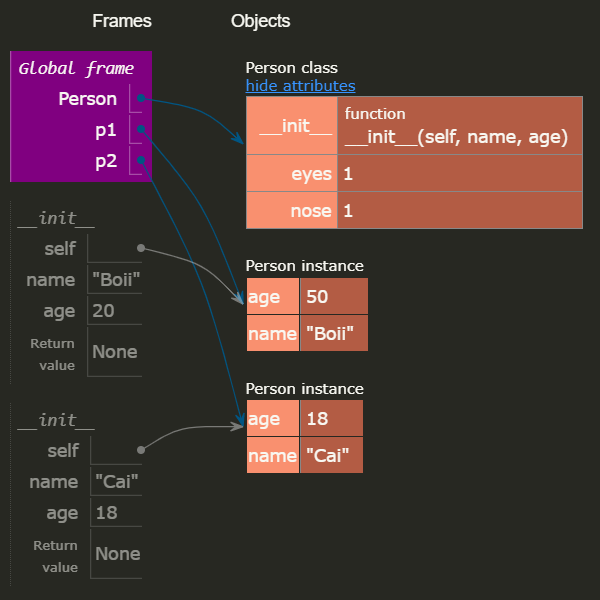class Person:
# 类变量、静态字段
eyes = 2

def __init__(self, name, age):
self.name = name
self.age = age

# 创建两个对象
p1 = Person('Boii', 20)
p2 = Person('Cai', 18)

# 通过类 访问静态变量
print(Person.eyes)        # 2
# 通过对象 访问静态变量
print(p1.eyes)       # 2
print(p2.eyes)       # 2

### 通过类 修改静态变量
Person.eyes = 1
# 再通过类 访问静态变量
print(Person.eyes)        # 1
# 再通过对象 访问静态变量
print(p1.eyes)       # 1
print(p2.eyes)       # 1

### 通过对象 修改对象变量
p1.eyes = 50

# 再通过类 访问静态变量
print(Person.eyes)        # 1
# 再通过对象 访问静态变量
print(p1.eyes)       # 50    此时personA的内存里已经有eyes这个变量了
print(p2.eyes)       # 1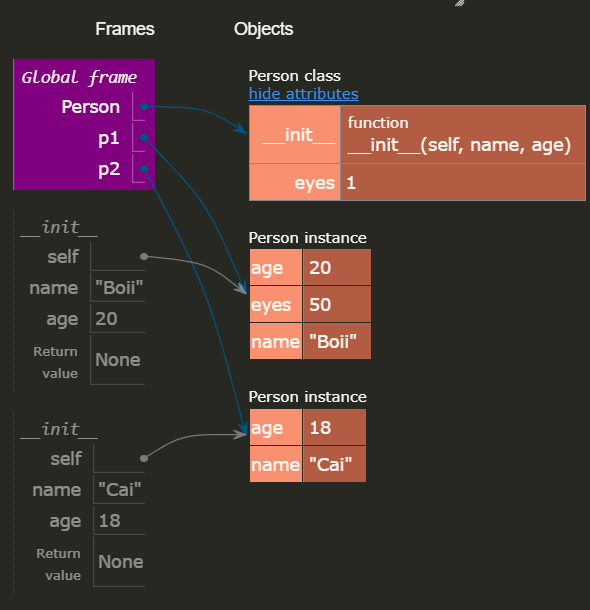#### 另外¶

class Person:
# 类变量、静态字段
eyes = 2
d = {1: 'A', 2: 'B'}

def __init__(self, name, age):
# 对象变量、普通字段
self.name = name
self.age = age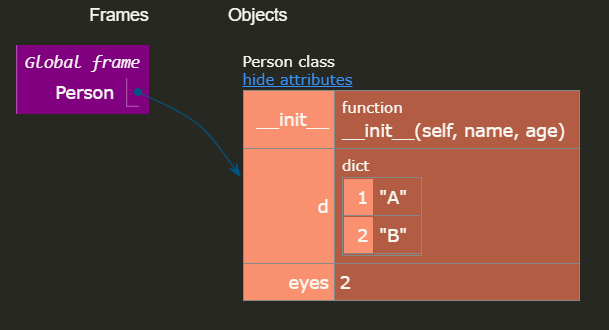# 创建两个对象
p1 = Person('Boii', 20)
p2 = Person('Cai', 18)

# 通过类 修改静态变量, 改动的是类空间里的eyes
Person.eyes = 1

# 通过对象 修改静态变量, 是复制一份eyes到对象空间里并修改
p1.eyes = 3

# 通过对象 修改静态字典变量, 并不会复制一份d到对象空间里
p1.d = 50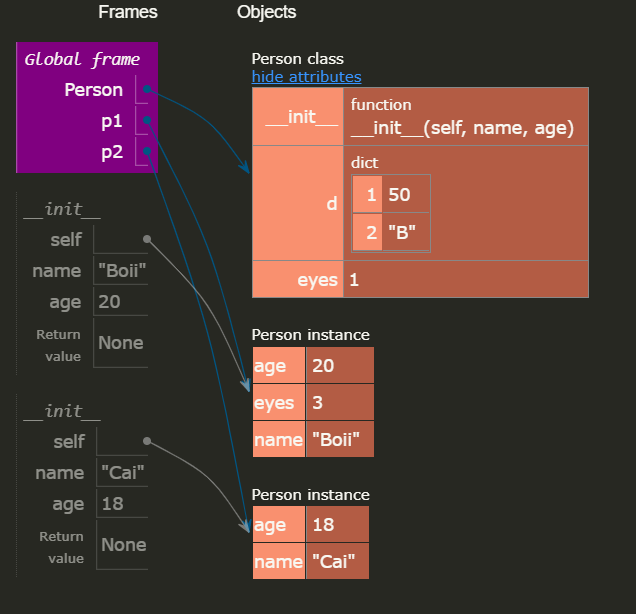#### 小结¶

1. 类变量，又称静态字段、类属性
2. 对象变量，又称普通字段、实例属性
3. 类变量是所有对象共有的，对象变量是对象自己独有的
4. 类变量可以通过 类名.类变量对象.类变量访问
5. 类变量可以通过 类名.类变量对象.类变量修改
6. 通过 对象.类变量 修改时，除非该类变量是字典类型，否则都会把类变量复制一份到对象中去

### 实例方法、类方法、静态方法¶

#### 实例方法¶

• 定义：第一个参数必须是实例对象，该参数名一般约定为“self”，通过它来传递实例的属性和方法（也可以传类的属性和方法）； 如p1.instanceMethod()，Python 解释器会把对象 p1 传给self参数
• 调用：只能由实例对象调用。
class Person:
...
...
# 定义实例方法
def walk(self, step):
return f"I walked {step} steps."

p1 = Person()
print(p1.walk(5))        # 调用实例方法

-------------------------------
Output:

I walked 5 steps.


#### 类方法 @classmethod¶

• 定义：使用装饰器@classmethod。第一个参数必须是当前类对象，该参数名一般约定为“cls”，通过它来传递类的属性和方法（不能传实例的属性和方法）；

p1.clsMethodPerson.clsMethod，Python解释器会把类 Person 传给 cls 参数

• 调用：类对象或实例对象都可以调用。

class Person:
__count = 0

def __init__(self):
Person.__count += 1
...
...
@classmethod
def get_count(cls):
return cls.__count


#### 静态方法 @staticmethod¶

• 定义：使用装饰器@staticmethod。参数随意，没有“self”和“cls”参数，但是方法体中不能使用类或实例的任何属性和方法；
• 调用：类对象或实例对象都可以调用。
class Person:
__count = 0

def __init__(self):
Person.__count += 1
...
...
@staticmethod
def get_time():
return time.time()


### 构造方法¶

def __init__(self)就是类的构造方法

self可以换成其他，但是为避免争议和歧义，最好用self

class Animal:
def __init__(self):
pass

animalA = Animal()    # self 不需要传入
animalA.weigth = 180  # 可以自由的增加对象属性

class Person:
def __init__(self, name, age):
pass

personA = Person('Boii', 20)    # 创建对象时必须给 name 和 age

personB = Person()    # ！！错误


### 访问限制¶

Example

# public 示例

class Person:
def __init__(self, name):
self.name = name    # public 属性

def show(self):
print(self.name)    # 类内部可以访问

person = Person('Boii')

print(person.name)    # Boii 类外部也可以访问

## Person.py
class Person:
def __init__(self, name):
self._name = name    # protected 属性

def show(self):
print(self._name)     # 当前类中可以访问

person = Person('Boii')

person.show()                 # Boii

## Student.py
from Person import Person

class Student(Person):
def __init__(self, name, age):
super().__init__(name)    # 在子类中通过 super().__init__() 访问
self.age = age

def show(self):
print(f'姓名：{self._name}, 年龄：{self.age}')

student = Student('Boii', 20)

student.show()            # 姓名：Boii, 年龄：20

# private 示例

class Person:
def __init__(self, name):
self.__name = name

def show(self):
print(self.__name)

def get_name(self):
return self.__name

def set_name(self, name):
self.__name = name

person = Person('Boii')

print(person.__name)        # 错误！！只能在类中访问私有变量

print(person.get_name())    # Boii, 通过get方法访问

person.set_name('Alice')    # 通过set方法改变

print(person.get_name())    # Alice


private变量实际上是因为 python解释器对外把 __name 改成了 _Person__name，依然可以通过person._Person__name来访问，但是强烈建议不要这么做。

## 封装、继承和多态¶

面向对象三大特性：封装、继承、多态

- **封装**

把一类东西共通的属性、行为定义在一个类中，就是封装。

- **继承**

一个类，继承了别的类以后，这个类叫做子类

被继承的类，叫做基类、父类、超类

继承以后，子类就拥有了父类的全部 非private 功能

Python中，子类可以同时继承多个父类

- **多态**

在子类中编写与父类同名的方法，叫做方法重写，

适用于父类功能不能满足子类要求时。这称之为**多态**

当父类子类的方法相同时，总是会优先调用子类的方法

class Animal:
def run(self):
print('Running---')

class Dog(Animal):    # 继承父类 Animal
pass

class Cat(Animal):    # 继承父类 Animal
def run(self):    # 方法重写
print('Cat is Running---')

dog = Dog()
dog.run()    # Running---

cat = Cat()
cat.run()    # Cat is Running---


### 继承¶

class ClsName(BaseClass)

#### 单继承¶

# 子类没有__init__，默认调用父类的__init__
# 父类的__init__没有参数，所以子类可以不写__init__

class Person:
def __init__(self):
self.name = "Anonymity"
self.age = 18

class Student(Person):
pass

s = Student()

# 父类的__init__有参数，子类必须有__init__，并调用父类的__init__且传参

class Person:
def __init__(self, name):
self.name = name

class Student(Person):
def __init__(self, name, age):
self.age = age
super().__init__(name)

s = Student("Boii", 18)


#### 多继承¶

• 如果子类没有__init__()，会调用第一个父类的__init__()
• 如果第一个父类没有__init__()，会找第二个父类，以此类推...

class BaseA:
def __init__(self, name, age):
self.name = name
self.age = age

class BaseB:
def __init__(self, gender, nationality):
self.gender = gender
self.nationality = nationality

class Student(BaseA, BaseB):
def __init__(self, name, age, gender, nationality):

BaseA(Student, self).__init__(name, age)

BaseB.__init__(self, gender, nationality)

s = Student("Boii", 18, "male", "China")


#### 钻石继承¶

     [Base]
↗     ↖
[A]      [B]
↖     ↗
[sub]


class Base:
def __init__(self):
print("Base.__init__")

class A(Base):
def __init__(self):
Base.__init__(self)
print("A.__init__")

class B(Base):
def __init__(self):
Base.__init__(self)
print("B.__init__")

class sub(A, B):
def __init__(self):
A.__init__(self)
B.__init__(self)
print("sub.__init__")

sub()

# Output:
Base.__init__
A.__init__
Base.__init__
B.__init__
sub.__init__

Base 的 __init__() 被调用了两次

class Base:
def __init__(self):
print("Base.__init__")

class A(Base):
def __init__(self):
super().__init__()
print("A.__init__")

class B(Base):
def __init__(self):
super().__init__()
print("B.__init__")

class sub(A, B):
def __init__(self):
super().__init__()
print("sub.__init__")

sub()

# Output:
Base.__init__
B.__init__
A.__init__
sub.__init__


### 多态的好处¶

Animal 是 Animal 类型；Dog 是 Dog 类型，也是 Animal 类型；Cat 同理

>>> animal = Animal()
>>> dog = Dog()
>>> cat = Cat()

>>> isinstance(animal, Animal)
True
>>> isinstance(dog, Dog)
True
>>> isinstance(dog, Animal)
True
>>> isinstance(cat, Cat)
True
>>> isinstance(cat, Animal)
True
>>> isinstance(animal, Dog)
False


def run_twice(animal):
animal.run()
animal.run()


>>> animal = Animal()
>>> dog = Dog()
>>> cat = Cat()

>>> run_twice(animal)
Running---
Running---

>>> run_twice(cat)
Cat is Running---
Cat is Running---

>>> run_twice(dog)
Running---
Running---


• 对扩展开放：允许新增Animal子类；
• 对修改封闭：不需要修改依赖Animal类型run_twice()等函数

#### 多态在静态语言和动态语言中的区别¶

class Timer:
def run(self):
print('Start---')


class Animal(object):   # 编写Animal类
def run(self):
print("Animal is running...")

class Dog(Animal):      # Dog类继承Amimal类，没有run方法
pass

class Cat(Animal):      # Cat类继承Animal类，有自己的run方法
def run(self):
print('Cat is running...')
pass

class Car(object):      # Car类不继承，有自己的run方法
def run(self):
print('Car is running...')

class Stone(object):    # Stone类不继承，也没有run方法
pass

def run_twice(animal):
animal.run()
animal.run()

run_twice(Animal())
run_twice(Dog())
run_twice(Cat())
run_twice(Car())
run_twice(Stone())


Animal is running...
Animal is running...
Animal is running...
Animal is running...
Cat is running...
Cat is running...
Car is running...
Car is running...

AttributeError: 'Stone' object has no attribute 'run'